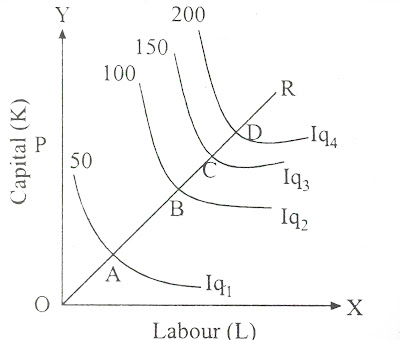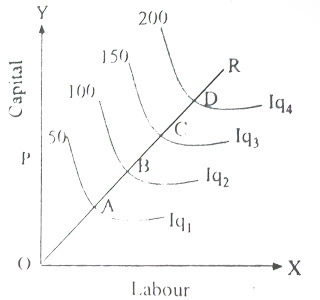### Law of Returns to Scale in Production

 Returns to scale describe the change in output when all inputs are changed/increased by the same proportion. This means that returns to scale explains production activities over the long run. Over the long run a firm can increase all inputs by equal proportion or by unequal proportion. Returns to scale is based on the assumption that over the long run all inputs of production are
increased by equal proportion. According to A. Koutsoyiannis, “The term returns to scale refers to the changes in output as all factors change by the same proportion.” This law of returns can also be defined as, “Returns to scale related to the behavior of total output as all inputs are varied and is a long run concept.”

When each of the inputs of production are increased proportionately, output may increases more than proportionately or less than proportionately or equi-proportionatly. If it increases more than the percentage increase in inputs, it is called increasing returns to scale (IRS); if output increases by less than the percentage increase in inputs it is called decreasing returns to scale (DRS); and if output increase by equal proportion to the increase in inputs, it is called constant returns to scale (CRS). The concept of returns to scale is summarized in table.
Table: Returns to Scale
 Increase in the Quantity  of Factors/inputs Increase in Output Type of Returns  to Scale 10% 20% Increasing 10% 10% Constant 10% 5% Decreasing
Increasing returns to scale occur when the economies of scale operate. Diminishing returns to scale occur when the diseconomies of scale operate. Constant returns to scale are an intermediary situation, when certain advantages of large scale production are counterbalanced by certain disadvantages.

The law of returns of scale can be explained with the help of Iso-quants for a single output with the use of two inputs.

## i) Increasing returns to Scale (IRS)

When a certain proportionate change in both the inputs K and L, leads to a more than proportionate change/increase in output, it exhibits increasing return to scale. For instance, if quantities of both the inputs, K and L are successively doubled and the corresponding output is more than doubled, the return to scale is to be increasing return. It is illustrated with the help of figure.Increasing Returns to Scale
The movement from point A to B as the line/ray OR should mean doubling the inputs K and L. In above figure, input combination increases from 1K + 1L to 2K + 2L. As a result of doubling the inputs, output is more than doubled. Similarly, the movement from point A to B, B to C, C to D indicates increase in inputs as a result of which the output increases more than the increase in inputs. Along the ray OR, the gap between AB, BC and CD is decreasing, that is OA > AB > BC > CD.

Increasing returns to scale (IRS) means the reduction in average cost of production with the expansion in the scale of the firm and increase in the size of output. That is, increasing returns to scale is another name of decreasing average cost of production with increase in the size of production.

Causes of increasing returns to scale
a) Specialization: Each worker can acquire specialization in the performance of simple repetitive task rather than many different tasks. As a result, labor productivity registers a rise.

b) Dimensional relation: Increasing returns to scale is a matter of dimensional relation. For example, when the size of cloths (10m x 20m = 200 sq. m) is doubled to 20m x 40m = 800 sq. m., the size of the cloth is more than doubled, 800 sq. m. is 4 times of 200 sq. m. Following this relationship, when labor and capita are doubled, output is more than doubled over initial level of output.

c) Use of specialized machinery: In addition, a large scale of operation permits the use of more productive specialized machinery, which was not possible at a smaller scale of operation.

d) Effect of research and development: Conduction of research and development (R & D) works modifies methods of production. Research and development bring efficiency, economy and effectiveness in the production process. Hence, more spending on research and development is possible only in firms of large size.

e) Use of indivisibilities: Capital equipment of a given capacity and entrepreneur skill are the indivisible factors. They cannot be sub-divided into parts. Hence, as output increases, there is better and effective utilization of these factors. Therefore, as a results of the effect of all these factors, a given proportionate increase in the amount of all inputs leads to more than proportionate increase in output.

## ii) Constant Returns to Scale (CRS)

When the change in output is proportional to the change in inputs, it exhibits constant return to scale (CRS). In other words, if quantities of both inputs, K and L are doubled and output is also doubled, then returns to scale is said to be constant.

A production function showing constant returns to scale is often called ‘liner and homogenous’ or homogeneous of the first degree’. The case of CRS is illustrated by means of figure.Constant Returns to Scale
If the figure, the movement from point A to B, B to C and C to D is assumed to indicate doubling of to the inputs. When inputs are doubled, output is also doubled. Similarly, the movement from A to C indicates trebling input, output also trebling. The gap between AB, BC and CD is same, that is OA = AB = BC = C which means that the distance of successive isoquants is equal in case of CRS.

The main reason for the operation of constant returns to scale is that beyond a certain point, internal and external economies are neutralized by the growing internal and external diseconomies of production. When inputs of same efficiency are duplicated output would increase by equal proportion.

## iii) Decreasing returns to scale (DRS)

 When output increases in a smaller proportion than the increase in all inputs, decreasing returns to scale (DRS) are said to operate. When a firm goes on expanding by increasing all its inputs/resources of production, eventually decreasing returns to scale will occur. For example, when inputs are doubled and output will less than doubled. The decreasing return to scale (IRS) is
illustrated with the help of figure.Decreasing Returns to Scale
When the inputs K and L are doubled, that is, when capital-labor combination is increased from 1K + 1L to 2K + 2L, output also increases but by less than the proportionate increase in inputs. The movement from A to B, B to C and C to D indicates increase in the inputs. But the output increases by less than the increase in inputs. The gap between AB, BC and CD is increasing, that is AB < BC < CD. When decreasing returns to scales applies, the distance of successive isoquants along the ray through origin increases. Decreasing returns to scale also means increase in average cost of production with the expansion in the size and output of the firm.

Causes of decreasing returns to scale
i) Managerial inefficiency: With the fast expansion of the scale of production, personal contacts and communication between (a) owners and management and (b) managers and labor, get rapidly reduced. Remote control and management replace close control and supervision. With the increase in managerial personnel/staff, decision-making becomes complex and delay in decision-making becomes inevitable. Implementation of decision is also delayed due to co-ordination problem between different sections and/or branches. As a result, output increases less than proportionately than the proportionate increase in inputs.

ii) Labor inefficiency: Another cause of decreasing returns to scale is over crowding of labor causing to loss of labor productivity and their accountability. On the other hand, increase in number of workers encourages labor union activities which mean simply the loss of output per unit of time.

iii) Exhaustible natural resources: The decreasing returns to scale may be found in the use of exhaustible natural resources. For example, if more and more fisherman used to fishing in a certain area of Trishuli river of Nepal, the catch of the fish will not increase in the same proportion.

iv) Fiscal diseconomies: With the expansion of the scale of production, the discount and concession that are available on bulk purchase of inputs come to end. As a result, output increases less proportion than the proportionate increase in all output.